# Python数据结构与时间复杂性## Big-O表示法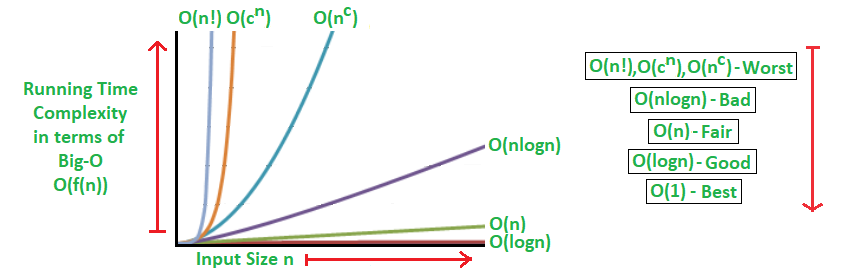• O(1)：无论您的集合有多大，执行操作所花费的时间都是恒定的。 这是恒定的时间复杂度符号。 这些操作尽可能快。 例如，检查集合内部是否有任何项目的操作是O(1)操作。
• O(log n)：当集合的大小增加时，执行操作所花费的时间对数增加。 这是对数时间复杂度表示法。 潜在优化的搜索算法为O(log n)。
• O(n)：执行操作所需的时间与集合中的项目数成线性正比。 这是线性时间复杂度符号。 就性能而言，这介于两者之间或中等。 作为一个实例，如果我们想对一个集合中的所有项目求和，那么我们将不得不遍历该集合。 因此，集合的迭代是O(n)操作。
• (n log n)：执行某项操作的性能是集合中项目数量的拟线性函数。 这称为准线性时间复杂度表示法。 优化排序算法的时间复杂度通常为n(log n)。
• O(n^2)：执行操作所需的时间与集合中项目的平方成正比。 这称为二次时间复杂度表示法。
• (n!)：当在操作中计算集合的每个单个排列时，因此执行操作所需的时间取决于集合中项目的大小。 这称为阶乘时间复杂度表示法。 非常慢。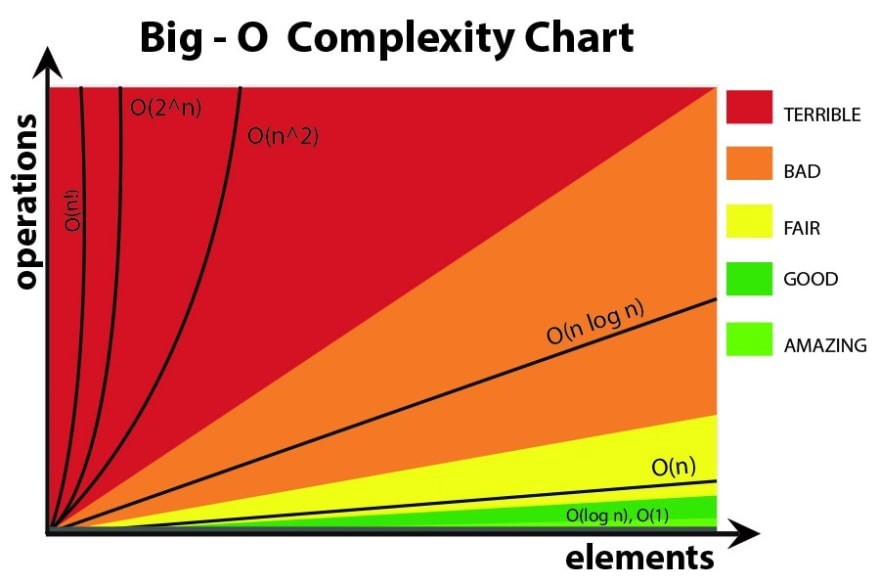### 最佳，平均，最差情况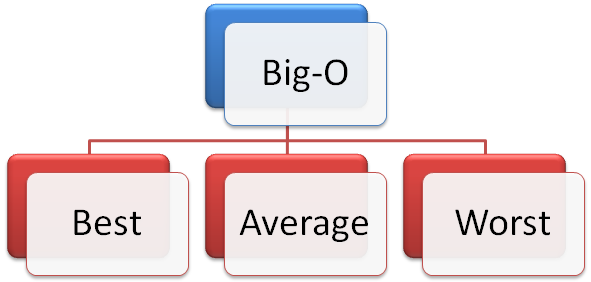• 最佳情况方案：顾名思义，这是当数据结构和集合中的项目以及参数处于最佳状态时的方案。 例如，假设我们要在集合中找到一个项目。 如果该项目恰好是集合的第一项，那么这是该操作的最佳情况。
• 平均情况是根据输入值的分布定义复杂度。
• 最坏的情况是可能需要一种操作，该操作需要在大型集合（例如列表）中找到位于最后一个项目的项目，并且算法会从第一个项目开始对集合进行迭代。

## Python内置方法的时间复杂度

### List列表

 操作 案例 类型 备注 Index l[i] O(1) Store l[i] = 0 O(1) Length len(l) O(1) Append l.append(5) O(1) Pop l.pop() O(1) l.pop(-1) 同理 Clear l.clear() O(1) l = [] 同理 Slice l[a:b] O(b-a) l[1:5]:O(l)/l[:]:O(len(l)-0)=O(N) Extend l.extend(…) O(len(…)) 取决于…的长度 Construction list(…) O(len(…)) 取决于…的长度 Insert l.insert(1) O(N) Pop l.pop(i) O(N) O(N-i): l.pop(0):O(N) (see above) check ==, != l1 == l2 O(N) Insert l[a:b] = … O(N) Delete del l[i] O(N) 取决于i的位置，最坏的情况为O(N) Containment x in/not in l O(N) 需逐个查找导致 Copy l.copy() O(N) l[:] 同理 Remove l.remove(…) O(N) Extreme value min(l)/max(l) O(N) 需逐个查找导致 Reverse l.reverse() O(N) Iteration for v in l: O(N) 当循环中没有return或break的时候，为O(N) Sort l.sort() O(N Log N) Multiply k*l O(k N)

### dict字典及defaultdict默认字典

 操作 案例 类型 备注 Index d[k] O(1) Store d[k] = v O(1) Length len(d) O(1) Delete del d[k] O(1) get/setdefault d.get(k) O(1) Pop d.pop(k) O(1) Pop item d.popitem() O(1) Clear d.clear() O(1) 与 s = {} 或 s = dict() 同理 View d.keys() O(1) 与 d.values() 同理 Construction dict(…) O(len(…)) Iteration for k in d: O(N) 包含以下方法： keys, values, items

### Set集合

 操作 案例 类型 备注 Length len(s) O(1) Add s.add(5) O(1) Containment x in/not in s O(1) 对比 list/tuple 的O(N) 快很多 Remove s.remove(..) O(1) compare to list/tuple – O(N) Discard s.discard(..) O(1) Pop s.pop() O(1) popped value “randomly” selected Clear s.clear() O(1) 与 s = set() 同理 Construction set(…) O(len(…)) 取决于 … 的长度 check ==, != s != t O(len(s)) 与len(t)同理 <=/< s <= t O(len(s)) 类似issubset方法 >=/> s >= t O(len(t)) Union s | t O(len(s)+len(t)) Intersection s & t O(len(s)+len(t)) Difference s – t O(len(s)+len(t)) Symmetric Diff s ^ t O(len(s)+len(t)) Iteration for v in s: O(N) 当循环中没有return或break的时候，为O(N) Copy s.copy() O(N)

### collections.deque双向队列

deque（double-ended queue，双向队列）是以双向链表的形式实现的 (Well, a list of arrays rather than objects, for greater efficiency)。双向队列的两端都是可达的，但从查找队列中间的元素较为缓慢，增删元素就更慢了。

 操作 案例 类型 备注 Copy dq.copy() O(n) append dq.append(5) O(1) appendleft dq.appendleft(1) O(1) 作用相当于 list.insert(0,1), 但后者效率为O(N) pop dq.pop() O(1) popleft dq.popleft() O(1) extend dq.extend(k) O(len(k)) extendleft dq.extendleft(k) O(len(k)) rotate dq.rotate(k) O(len(k)) remove dq.remove(1) O(n)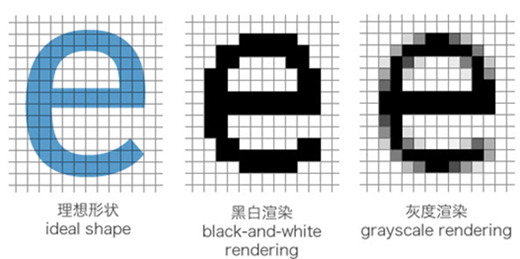##### 网站与APP开发中的字体设置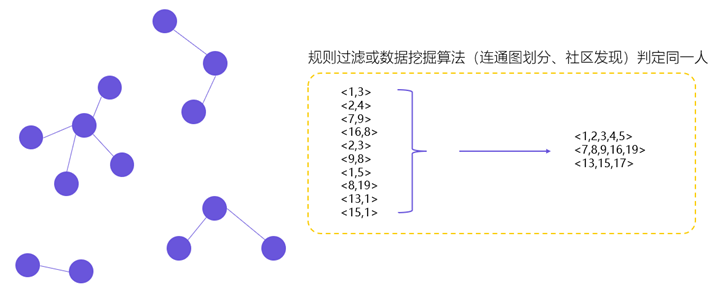##### 用户体系搭建之ID-Mapping